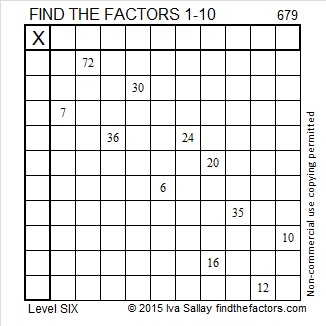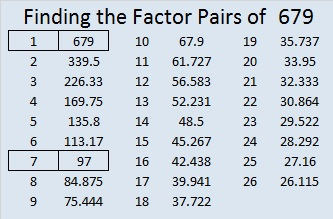# 679 and Level 6

679 is the sum of the three primes from 223 to 229 and the sum of the nine primes from 59 to 97.

Since 97 is one of its factors, 679 is the hypotenuse of the Pythagorean triple 455-504-679. What is the greatest common factor of those three numbers?Print the puzzles or type the solution on this excel file: 10 Factors 2015-11-09

—————————————————————————————————

• 679 is a composite number.
• Prime factorization: 679 = 7 x 97
• The exponents in the prime factorization are 1 and 1. Adding one to each and multiplying we get (1 + 1)(1 + 1) = 2 x 2 = 4. Therefore 679 has exactly 4 factors.
• Factors of 679: 1, 7, 97, 679
• Factor pairs: 679 = 1 x 679 or 7 x 97
• 679 has no square factors that allow its square root to be simplified. √679 ≈ 26.057628.—————————————————————————————————This site uses Akismet to reduce spam. Learn how your comment data is processed.